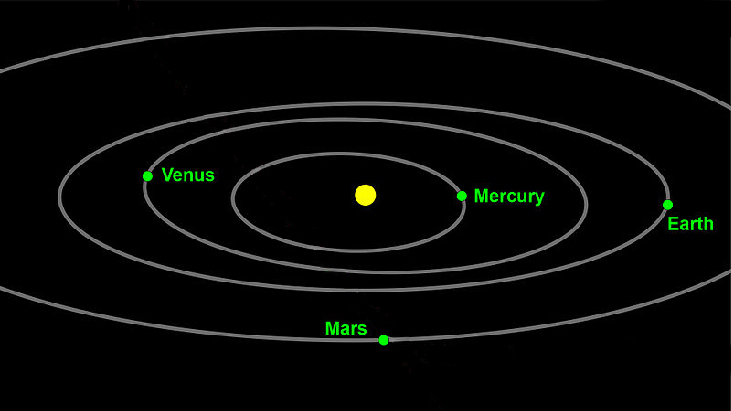# 10.4 Polar coordinates: graphs

 Page 1 / 16
In this section you will:
• Test polar equations for symmetry.
• Graph polar equations by plotting points.

The planets move through space in elliptical, periodic orbits about the sun, as shown in [link] . They are in constant motion, so fixing an exact position of any planet is valid only for a moment. In other words, we can fix only a planet’s instantaneous position. This is one application of polar coordinates    , represented as $\text{\hspace{0.17em}}\left(r,\theta \right).\text{\hspace{0.17em}}$ We interpret $\text{\hspace{0.17em}}r\text{\hspace{0.17em}}$ as the distance from the sun and $\text{\hspace{0.17em}}\theta \text{\hspace{0.17em}}$ as the planet’s angular bearing, or its direction from a fixed point on the sun. In this section, we will focus on the polar system and the graphs that are generated directly from polar coordinates.Planets follow elliptical paths as they orbit around the Sun. (credit: modification of work by NASA/JPL-Caltech)

## Testing polar equations for symmetry

Just as a rectangular equation such as $\text{\hspace{0.17em}}y={x}^{2}\text{\hspace{0.17em}}$ describes the relationship between $\text{\hspace{0.17em}}x\text{\hspace{0.17em}}$ and $\text{\hspace{0.17em}}y\text{\hspace{0.17em}}$ on a Cartesian grid, a polar equation describes a relationship between $\text{\hspace{0.17em}}r\text{\hspace{0.17em}}$ and $\text{\hspace{0.17em}}\theta \text{\hspace{0.17em}}$ on a polar grid. Recall that the coordinate pair $\text{\hspace{0.17em}}\left(r,\theta \right)\text{\hspace{0.17em}}$ indicates that we move counterclockwise from the polar axis (positive x -axis) by an angle of $\text{\hspace{0.17em}}\theta ,\text{\hspace{0.17em}}$ and extend a ray from the pole (origin) $\text{\hspace{0.17em}}r\text{\hspace{0.17em}}$ units in the direction of $\text{\hspace{0.17em}}\theta .\text{\hspace{0.17em}}$ All points that satisfy the polar equation are on the graph.

Symmetry is a property that helps us recognize and plot the graph of any equation. If an equation has a graph that is symmetric with respect to an axis, it means that if we folded the graph in half over that axis, the portion of the graph on one side would coincide with the portion on the other side. By performing three tests, we will see how to apply the properties of symmetry to polar equations. Further, we will use symmetry (in addition to plotting key points, zeros, and maximums of $\text{\hspace{0.17em}}r\right)\text{\hspace{0.17em}}$ to determine the graph of a polar equation.

In the first test, we consider symmetry with respect to the line $\text{\hspace{0.17em}}\theta =\frac{\pi }{2}\text{\hspace{0.17em}}$ ( y -axis). We replace $\text{\hspace{0.17em}}\left(r,\theta \right)\text{\hspace{0.17em}}$ with $\text{\hspace{0.17em}}\left(-r,-\theta \right)\text{\hspace{0.17em}}$ to determine if the new equation is equivalent to the original equation. For example, suppose we are given the equation $\text{\hspace{0.17em}}r=2\mathrm{sin}\text{\hspace{0.17em}}\theta ;$

This equation exhibits symmetry with respect to the line $\text{\hspace{0.17em}}\theta =\frac{\pi }{2}.$

In the second test, we consider symmetry with respect to the polar axis ( $\text{\hspace{0.17em}}x$ -axis). We replace $\text{\hspace{0.17em}}\left(r,\theta \right)\text{\hspace{0.17em}}$ with $\text{\hspace{0.17em}}\left(r,-\theta \right)\text{\hspace{0.17em}}$ or $\text{\hspace{0.17em}}\left(-r,\pi -\theta \right)\text{\hspace{0.17em}}$ to determine equivalency between the tested equation and the original. For example, suppose we are given the equation $\text{\hspace{0.17em}}r=1-2\mathrm{cos}\text{\hspace{0.17em}}\theta .$

The graph of this equation exhibits symmetry with respect to the polar axis.

In the third test, we consider symmetry with respect to the pole (origin). We replace $\text{\hspace{0.17em}}\left(r,\theta \right)\text{\hspace{0.17em}}$ with $\text{\hspace{0.17em}}\left(-r,\theta \right)\text{\hspace{0.17em}}$ to determine if the tested equation is equivalent to the original equation. For example, suppose we are given the equation $\text{\hspace{0.17em}}r=2\mathrm{sin}\left(3\theta \right).$

$\begin{array}{c}\text{\hspace{0.17em}}\text{\hspace{0.17em}}\text{\hspace{0.17em}}\text{\hspace{0.17em}}\text{\hspace{0.17em}}r=2\mathrm{sin}\left(3\theta \right)\\ -r=2\mathrm{sin}\left(3\theta \right)\end{array}$

The equation has failed the symmetry test , but that does not mean that it is not symmetric with respect to the pole. Passing one or more of the symmetry tests verifies that symmetry will be exhibited in a graph. However, failing the symmetry tests does not necessarily indicate that a graph will not be symmetric about the line $\text{\hspace{0.17em}}\theta =\frac{\pi }{2},\text{\hspace{0.17em}}$ the polar axis, or the pole. In these instances, we can confirm that symmetry exists by plotting reflecting points across the apparent axis of symmetry or the pole. Testing for symmetry is a technique that simplifies the graphing of polar equations, but its application is not perfect.

#### Questions & Answers

write down the polynomial function with root 1/3,2,-3 with solution
if A and B are subspaces of V prove that (A+B)/B=A/(A-B)
write down the value of each of the following in surd form a)cos(-65°) b)sin(-180°)c)tan(225°)d)tan(135°)
Prove that (sinA/1-cosA - 1-cosA/sinA) (cosA/1-sinA - 1-sinA/cosA) = 4
what is the answer to dividing negative index
In a triangle ABC prove that. (b+c)cosA+(c+a)cosB+(a+b)cisC=a+b+c.
give me the waec 2019 questions
the polar co-ordinate of the point (-1, -1)
prove the identites sin x ( 1+ tan x )+ cos x ( 1+ cot x )= sec x + cosec x
tanh`(x-iy) =A+iB, find A and B
B=Ai-itan(hx-hiy)
Rukmini
what is the addition of 101011 with 101010
If those numbers are binary, it's 1010101. If they are base 10, it's 202021.
Jack
extra power 4 minus 5 x cube + 7 x square minus 5 x + 1 equal to zero
the gradient function of a curve is 2x+4 and the curve passes through point (1,4) find the equation of the curve
1+cos²A/cos²A=2cosec²A-1
test for convergence the series 1+x/2+2!/9x3ByByByBy